﻿ Van der Waerden numbers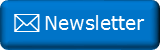Newsletter: Click here to join an email list and be contacted when a new article is published.      Advertisement

# Van der Waerden numbers

Van der Waerden's theorem is a theorem in the branch of mathematics called Ramsey theory.

It relates to ways that collections can be coloured, in order, avoiding spacing of colours that are a defined length arithmetic progression apart. They are named after the Dutch mathematician B. L. van der Waerden. In pure form they can be described as W(c,k), where c represents the number of colours in the sequence, and k represents the number of elements in the arithmetic progression.

The simplest (non-trivial) example is W(2,3)

In the diagram below you can see there are currently eight boxed shaded with two colours (Red and Blue) c=2.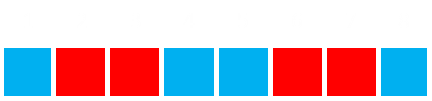Above, there are no sequences of either blue or red that form a three step k=3 chain.

If a ninth element were to be added to the end of the sequence, if it were coloured red, then cells 3,6,9 would be a three chain arithmetic sequence. If it were coloured blue, then 1,5,9 would be in progression.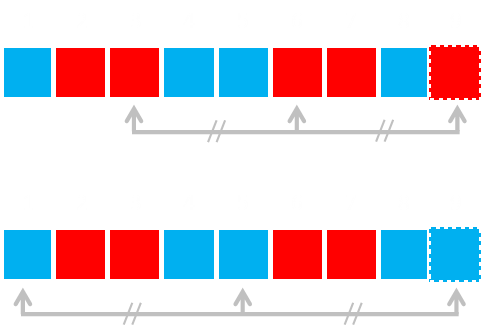There is no way of coloring 1 through 9 without creating a three step progression of one colour or another, so W(2,3)=9

The above sequence is not unique; there are six solutions (which includes the rotations, reflections and complements).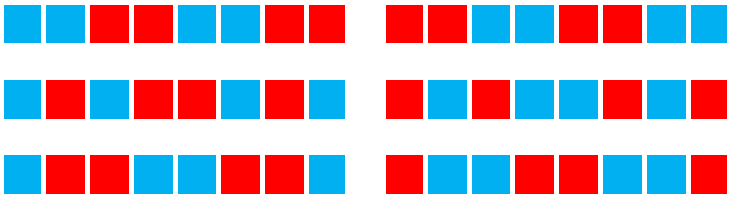## W(3,3)

If we add another colour, and keep the max sequenece to three, then the length increases to 27.

There are 48 solutions to W(3,3). A couple are shown below: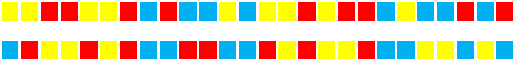## W(2,4)

If we keep at two colours, and increase the max length of the arithmetic prgression to four then the length increases to 35.

There are 28 solutions to W(2,4). A couple are shown below:## W(2,5)

Increasing the progression length to five, the value of W(2,5)=178

Here is one solution in string form:

121112122212112111121121222121112222122221222211121222111121111211212221211122221222212222111212221211211112111122212111222212222122221112122211112111121111222121112122122221221

## W(2,6)

Increasing the progression length to six, the value of W(2,6)=1,132

Here is one solution in string form:

221111221222221211112111221111121121112122211121221212211221121211212221112122212212222211222122221211111211222212122221121111121222212221122222122122212111222121121211221122121221211122212111211211111221112111121222221221111222111122122222121111211122111112112111212221112122121221122112121121222111212221221222221122212222121111121122221212222112111112122221222112222212212221211122212112121122112212122121112221211121121111122111211112122222122111122211112212222212111121112211111211211121222111212212122112211212112122211121222122122222112221222212111112112222111222211211111212222122211222221221222121112221211212112211221212212111222121112112111112211121111212222212211112221111221222221211112111221111121121112122211121221212211221121211212221112122212212222211222122221211111211222212122221121111121222212221122222122122212111222121121211221122121221211122212111211211111221112111121222221221111222111122122222121111211122111112112111212221112122121221122112121121222111212221221222221122212222121111121122221212222112111112122221222112222212212221211122212112121122112212122121112221211121121111122111211112122222122111121

## All known

Below is a table of the known Van der Waerden numbers.

There are currently only seven non-trivial numbers known. For the others, lower bounds have been determined, even though values are not currently known.

k c=2 c=3 c=4 c=5 c=6
3 9   27   76   >170   >223
4 35   293   >1,048   >2,254   >9,778
5 178   >2,173   >17,705   >98,740   >98,748
6 1,132   >11,191   >91,331   >540,025   >816,981
7 >3,703   >48,811   >420,217   >1,381,687   >7,465,909
8 >11,495   >238,400   >2,388,317   >10,743,258   >57,445,718
9 >41,265   >932,745   >10,898,729   >79,706,009   >458,062,329
10 >103,474   >4,173,724   >76,049,218   >542,694,970   >2,615,305,384
11 >193,941   >18,603,731   >305,513,57   >2,967,283,511   >3,004,668,671

## Off Diagonal Waerden numbers

All the examples above have been symmetric across the colours. For instance for W(3,3), each of the colours has the same restriction of not having a sequence of more the three in arithmetic progression. For off diagonal numbers, each colour can have in individual restriction on progression length e.g. W(3; 3,3,4). Here the first colour can have no number than three elements in a progression, the second three, and the third four.

Here are a couple of examples, with a sample for each:

W(3; 3,3,4)=51
22132333113323312322123112323313331133322131223231

W(3; 2,4,4)=40
332332322233222323313323222332223233233

W(3; 2,2,3,4)=25
443344433434214434433443

W(2; 3,8)=58
222212212222121222212221222221122222122212222121222212212

W(2; 4,5)=55
222122122221112112111222212212222111211211122221221222

W(6; 2,2,2,2,3,4)=33
65566645565663665612556566655666

## Other things

If you found this subject interesting, you might also like these other articles:De Bruijn Sequences. These are sequences of numbers that rotate through all possible combinations of the digits by sliding a window over them.Golomb Rulers and Costas Arrays. Golomb rulers are efficient rulers with minimal markings that allow measurement of many lengths by distances between various sets of points.Langford Sequences. These are sequences of numbers with distinct gaps between pairs in the sequence.And of course there is the venerable Eight Queens Problem; How to place eight queens on a chess board so that none are attacking another.

You can find a complete list of all the articles here.Click here to receive email alerts on new articles.